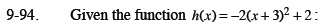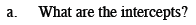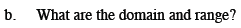Home > CCA2 > Chapter 9 > Lesson 9.3.2 > Problem9-94

9-94.
1. Given the function h(x) = −2(x + 3)2 + 2: Homework Help ✎

1. What are the intercepts?

2. What are the domain and range?Solve: 0 = −2(x + 3)2 + 2 and y = −2(0 + 3)2 + 2Domain: Are there any values that when input for x result in an undefined value for h(x)?
If not, the domain is all real numbers.

Range: Think about the shape of the graph.
Does it extend all the way up and all the way down, or does it have a highest or lowest point?